Échale un vistazo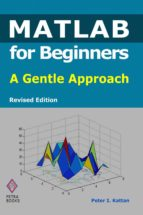# MATLAB FOR BEGINNERS EBOOK

## PETER I. KATTAN

, 2013
• Formato: EPUB - DRM
• Editorial: BOOKBABY
• Lengua: INGLÉS
• Año edición: 2013
• ISBN: 9781483515922
This book is written for beginners and students who wish to learn MATLAB. One of the objectives of writing this book is to introduce MATLAB to students in high schools. The material presented is very easy and simple to understand -- written in a gentle manner. The topics covered in the book include arithmetic operations, variables, mathematical functions, complex numbers, vectors, matrices, programming, graphs, solving equations, and an introduction to calculus. In addition, the MATLAB Symbolic Math Toolbox is emphasized in this book. There are also over 230 exercises at the ends of chapters for students to practice. Detailed solutions to all the exercises are provided in the second half of the book. The author has been using MATLAB for the past fifteen years and is the author of the best selling book "MATLAB Guide to Finite Elements". This description applies to the Revised Edition.

\$199.00

\$189.05

\$189.05

Inseparables, comprar "MATLAB FOR BEGINNERS (EBOOK)" junto con: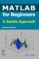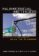NUMERICAL METHODS (EBOOK)

Cómpralos hoy por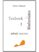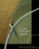LINEAR SYSTEMS THEORY (EBOOK)

Cómpralos hoy por

Datos del producto

• Editorial: BOOKBABY
• Lengua: INGLÉS
• ISBN: 9781483515922
• Año edición: 2013
• Formato: EPUB - DRM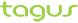Resumen

This book is written for beginners and students who wish to learn MATLAB. One of the objectives of writing this book is to introduce MATLAB to students in high schools. The material presented is very easy and simple to understand -- written in a gentle manner. The topics covered in the book include arithmetic operations, variables, mathematical functions, complex numbers, vectors, matrices, programming, graphs, solving equations, and an introduction to calculus. In addition, the MATLAB Symbolic Math Toolbox is emphasized in this book. There are also over 230 exercises at the ends of chapters for students to practice. Detailed solutions to all the exercises are provided in the second half of the book. The author has been using MATLAB for the past fifteen years and is the author of the best selling book "MATLAB Guide to Finite Elements". This description applies to the Revised Edition.

0

Valoración Media

Todavía no ha sido valorado

Valoraciones usuarios

• (0)
• (0)
• (0)
• (0)
• (0)

Recomendaciones usuarios

• 0% No ha sido todavía recomendado
Haz tu recomendación

## Opiniones "MATLAB FOR BEGINNERS (EBOOK)"

No hay opiniones para este producto

Hazte un hueco en la comunidad de Casa del Libro, regístrate